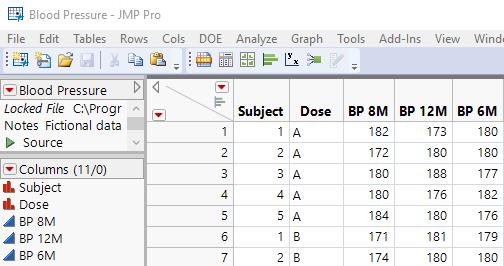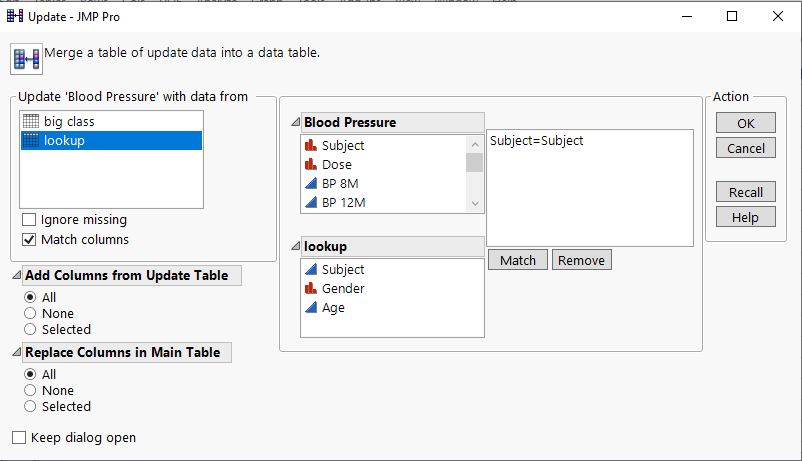Choose Language Hide Translation Bar
Highlighted

## Formulas similar to Frequently used excel formulas in JMP

How to enter a formula similar to sumif, count if and vlookup in jmp

Highlighted

## Re: Formulas similar to Frequently used excel formulas in JMP

Excel has many functions that are geared towards a Cell based spreadsheet.  A JMP data table is of an analytical format and has functions and platforms geared towards a Column based structure.  Each form has it's advantages, and each can somewhat mimic the others form.  That said, here is how I approach Countif, Sumif and Vlookup in JMP.

Given a simple Blood Pressure data table (This is subset of the Blood Pressure data table in the JMP Example tables):Countif

To count the number of blood pressure reading that each patient has that is over 180, the following formula structure is what I use

``Sum( :BP 8M > 180, :BP 12M > 180, :BP 6M > 180 )``

Countif has the nice feature of being able to specify a range of cells, but on the other hand, the JMP form allows for each column specified to potentially count based upon different criteria

``Sum( :BP 8M > 180, :BP 12M > 190, :BP 6M > 200 )``

Each of the Boolean comparisons will return a 1 if true and a 0 if false.

And the formula is automatically applied to each row automatically....no copy and paste to each row

Sumif

The way to handle this is just an expansion on the JMP solution for the Countif.  Instead of counting the Boolean values, one just places what value is to be added into an IF() function

``````Sum(If( :BP 8M > 180, :BP 8M, . ),If( :BP 12M > 180, :BP 8M, . ),
If( :BP 6M > 180, :BP 6M, . ))``````

This can get lengthy, and if that is what is needed, one can bring in For() loop(s) and column referencing.  Remember, JMP is a complete language and the formulas can virtually be of an complexity.

Vlookup

In my opinion, the way to do a Vlookup in JMP is a far easier method.  What is used, is the Update platform

Tables==>Update

It can do the same function as the Vlookup, or it can easily bring in multiple data columns, based upon multiple matches, such as matching not just on Subject but lets say, on Subject and Dose.  Interactively,Attached are two data tables.  The first one is the blood pressure table with all of the formulas and Update applied.  The second is the Lookup table referenced in the Update platform.

I have also added a couple of other formulas.  If you want to Countif or Sumif across rows,  a different methodology is used.

Jim
Article Labels

There are no labels assigned to this post.